Affine Transform
Not logged in

Back to main 4.3.0 Wiki page

## Affine Transformations

Starting with version 4.3.0 SpatiaLite supports several new SQL functions based on affine transformations,
Understanding and mastering all the underlying mathematics could easily be a rather difficult task, especially if you have absolutely no familiarity with this kind of operation.
So we'll start slowly and simply by first introducing a very simple practical example based on a joke about the geography of Italy and Sicily.

## Fancy Geography and amusing Math

### Preparing to start

(it simply corresponds to a slightly modified vector map of Italy's Regions as originally released by ISTAT under CC-BY license terms).
2. unzip the downloaded dataset and import the Shapefile into a SpatiaLite DB file. The appropriate import settings are shown in the side figure:
SRID=32632, charset=CP1252.
3. Extract Sicily into a separate database table by executing the following SQL statements:
```CREATE TABLE sicilia AS
SELECT * FROM italy WHERE cod_reg = 19;

SELECT RecoverGeometryColumn('sicilia', 'geometry', 32632, 'MULTIPOLYGON', 'XY');

DELETE FROM italy WHERE cod_reg = 19;
```
4. that's all: you are now ready to start this funny (and certainly not serious) tutorial about Affine Transformations.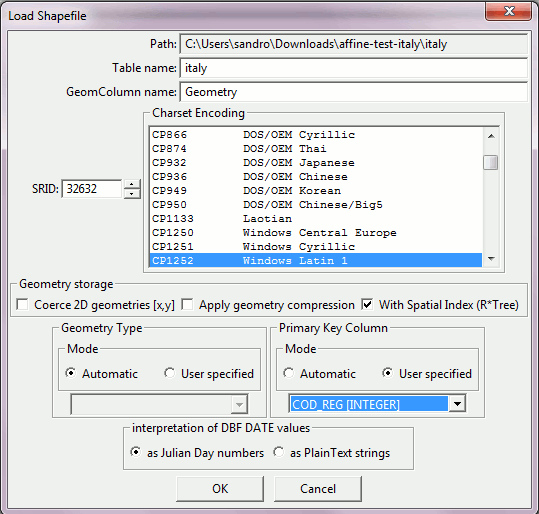### Preface

Look at a map of Italy; it appears obvious at first glance that Sicily is located in a very inconvenient position:
1. Sicily is placed too far South and too much close to North Africa; this implies an unpleasant torrid summer, and this later poses in turn many severe limitations to agricultural activities.
2. The Strait of Messina is exaggeratedly narrow, thus posing severe safety risks to the navigation of capital ships, big container ships, cruise liners and supertankers.
Even worse, from time to time some crazy politician starts strongly advocating the very stupid idea to build an incredibly costly bridge crossing the Strait, completely overlooking the very high seismic risk of this district and deliberately forgetting that in 1908 both towns of Messina and Reggio Calabria were completely destroyed by an earthquake followed by a tsunami.
More than 120,000 peoples lost their lives, and it was one of the most frightening natural disasters registered in Europe during modern times.
So we'll immediately start a theoretical case study intended to identify a possible alternative location for Sicily.
Incidentally we'll use the new SQL functions based on Affine Transformations for this task.
Let's go on.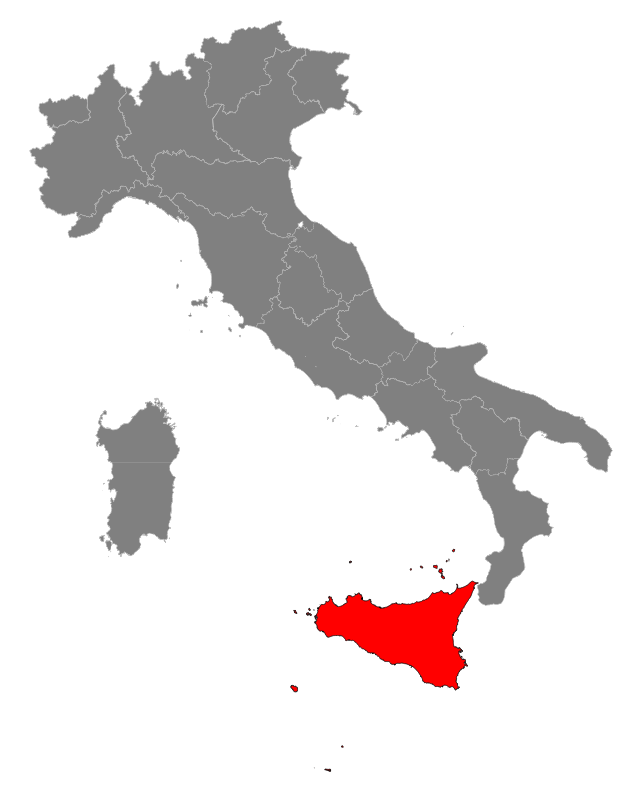### Step #1: translating Sicily into a more convenient position

There is plenty of free room in the Lower Tyrrhenian Sea, so we'll start by applying a translation to Sicily: this practically means adding (or subtracting) a constant value to the coordinates on both x and y axes.
After a very quick examination moving Sicily 150 km (i.e. 150,000 m) westward and 150 km northward seems to be an absolutely reasonable choice.
So using the Affine Transformation SQL functions we'll duly execute the following SQL statement:
```CREATE TABLE sicilia_1 AS
SELECT cod_reg,
ATM_Transform(geometry,
ATM_CreateTranslate(-150000, 150000)) AS geom
FROM sicilia;

SELECT RecoverGeometryColumn('sicilia_1', 'geom', 32632, 'MULTIPOLYGON', 'XY');
```
Remarks:
• all affine transformation related SQL function names start with an ATM_ prefix: this simply stands for Affine Transformation Matrix.
• ATM_Transform() is very similar to ST_Transform(); the most obvious difference is in that all transformation arguments are now expected to be passed in the form of an appropriate BLOB-serialized Affine Transformation Matrix.
• ATM_CreateTranslate() simply is an SQL function returning a BLOB-serialized Affine Transformation Matrix initialized in such a way to represent a simple 2D Translate accordingly to (tx, ty) arguments.
You could eventually define a complete 3D Translate by passing (tx, ty, tz) arguments, but this isn't strictly required in our current example.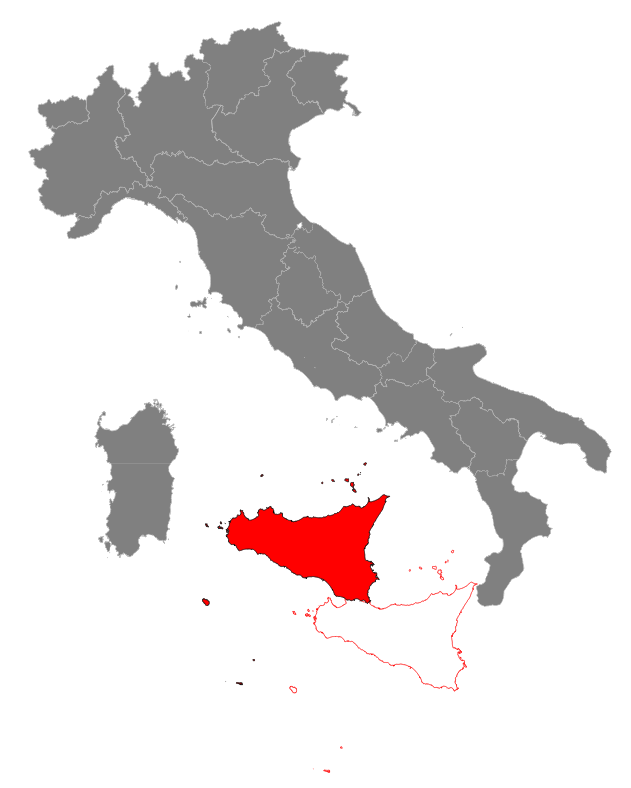### Step #2: rotating Sicily so to get a nice horizontal alignment

Aligning the southern shores of Sicily to an almost horizontal line will surely lead to a more nicely ordered layout: so we have now to apply a counterclockwise rotation of about 25.0 degrees.
Thanks to Affine Transformations, we can combine both the previous translation and the current rotation into a single movement (a so called rototranslation).
We simply have to execute the following SQL statement:
```CREATE TABLE sicilia_2 AS
SELECT cod_reg,
ATM_Transform(geometry,
ATM_Translate(
ATM_Translate(
ATM_Rotate(
ATM_CreateTranslate(-cx, -cy),
25),
cx, cy),
-150000, 150000)
) AS geom
FROM (SELECT cod_reg, ST_X(centroid) AS cx, ST_Y(centroid) AS cy, geometry
FROM (SELECT cod_reg, ST_Centroid(geometry) AS centroid, geometry
FROM sicilia) AS g1
) AS g2;

SELECT RecoverGeometryColumn('sicilia_2', 'geom', 32632, 'MULTIPOLYGON', 'XY');
```
Remarks:
• correctly handling Rotate is a little bit more difficult. Any rotation will always imply a fixed center point, and by default this is exactly placed at the coordinates origin: (0, 0).
• so a very naive attempt to directly invoke ATM_Rotate(25) will simply relocate Sicily in Southern Spain, and this absolutely isn't our intention.
• what we really need is a more complex sequence of chained transformations:
1. we'll start first by creating a new BLOB-Matrix intended to relocate Sicily's centroid exactly on the coordinates origin: ATM_CreateTranslate(-cx, -cy)
2. now we can safely chain the intended Rotate: ATM_Rotate(atm-blob, 25)
3. after applying Rotate we have now to restore the initial position: ATM_Translate(atm-blob, cx, cy)
4. and finally we'll apply the Translation intended to reposition Sicily westward and northward: ATM_Translate(atm-blob, -150000, 150000)
we've used two separate translations simply for didactic clarity: we could easily merge both them into a single translation:
ATM_Translate(atm-blob, cx - 150000, cy + 150000)
5. this way ATM_Transform() will receive the final BLOB-Matrix resulting by chaining all the above transformation steps in the correct sequence.
• Very important notice: Affine Transformations are not commutative: the relative order of subsequent operations in a complex transformation chain should always be very carefully considered.
• all the FROM (SELECT ... FROM (SELECT ...) AS g1) AS g2 stuff simply is a rather trivial SQL trick based on two nested sub-queries.
It's intended scope is simply to avoid multiple calls to ST_Centroid(), ST_X() and ST_Y() in order to get the centroid coordinates.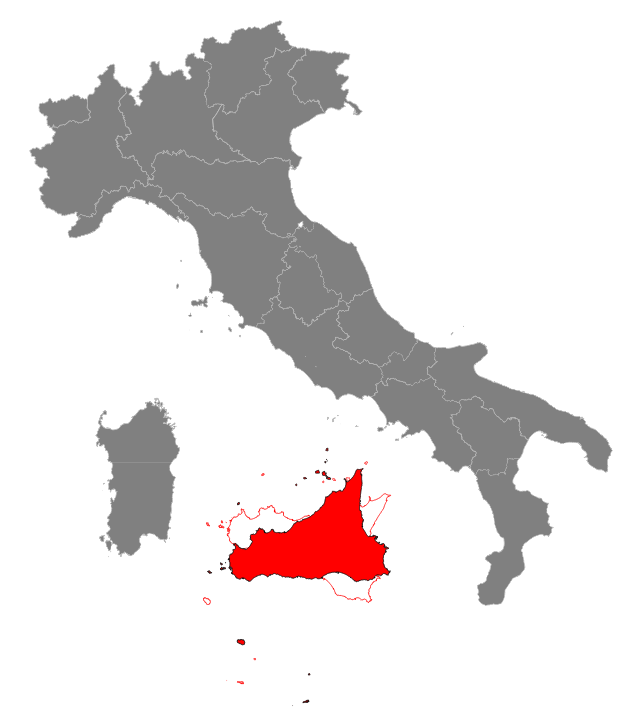### Step #3: inflating and reshaping Sicily

An increased surface is surely welcome, because it automatically implies more agricultural lands: on the other hand shortening a little bit the length of the southern coastline will surely facilitate mobility and communications.
So we'll now apply a scaling transformation using two different values: sx=0.9 and sy=1.3.
Once again, Affine Transformations enable us to combine altogether both translate, rotate and scale into a single transformation.
This is the corresponding SQL statement:
```CREATE TABLE sicilia_3 AS
SELECT cod_reg,
ATM_Transform(geometry,
ATM_Translate(
ATM_Translate(
ATM_Scale(
ATM_Rotate(
ATM_CreateTranslate(-cx, -cy),
25),
0.9, 1.3),
cx, cy),
-150000, 150000)
) AS geom
FROM (SELECT cod_reg, ST_X(centroid) AS cx, ST_Y(centroid) AS cy, geometry
FROM (SELECT cod_reg, ST_Centroid(geometry) AS centroid, geometry
FROM sicilia) AS g1
) AS g2;

SELECT RecoverGeometryColumn('sicilia_3', 'geom', 32632, 'MULTIPOLYGON', 'XY');
```
Remarks:
• there is nothing really new in this: we'll simply chain yet another transformation in the appropriate sequence order.
• ATM_Scale(atm-blob, sx, sy) is intended for the simpler 2D case.
• in the more general 3D case you can invoke ATM_Scale(atm-blob, sx, sy, sz)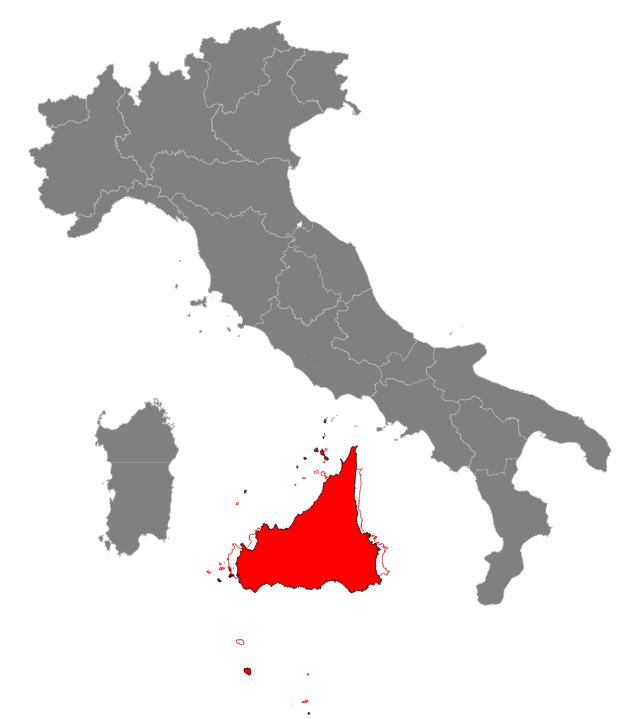### Step #4: final touch: reflecting Sicily

A reflected Sicily presents many interesting advantages: we'll examine all them in full detail in the final conclusions of the present study.
So we'll now apply a final reflection transformation; this simply corresponds to a 180 degrees rotation around the Y axis.
And the following is the final SQL statement applying all the above transformations in a single shot:
```CREATE TABLE sicilia_4 AS
SELECT cod_reg,
ATM_Transform(geometry,
ATM_Translate(
ATM_Translate(
ATM_YRoll(
ATM_Scale(
ATM_Rotate(
ATM_CreateTranslate(-cx, -cy),
25),
0.9, 1.3),
180),
cx, cy),
-150000, 150000)
) AS geom
FROM (SELECT cod_reg, ST_X(centroid) AS cx, ST_Y(centroid) AS cy, geometry
FROM (SELECT cod_reg, ST_Centroid(geometry) AS centroid, geometry
FROM sicilia) AS g1
) AS g2;

SELECT RecoverGeometryColumn('sicilia_4', 'geom', 32632, 'MULTIPOLYGON', 'XY');
```
Remarks:
• there are several possible rotations supported by Affine Transformation:
1. ATM_Rotate() always intends a 2D rotation, i.e. a rotation centered around the Z axis
2. In the more general 3D case there are three possible rotations, one for each axis.
3. the corresponding SQL functions are: ATM_XRoll(), ATM_YRoll() and ATM_ZRoll()
4. Note: ATM_ZRoll() and ATM_Rotate() simply are two different alias-names for the same identical SQL function.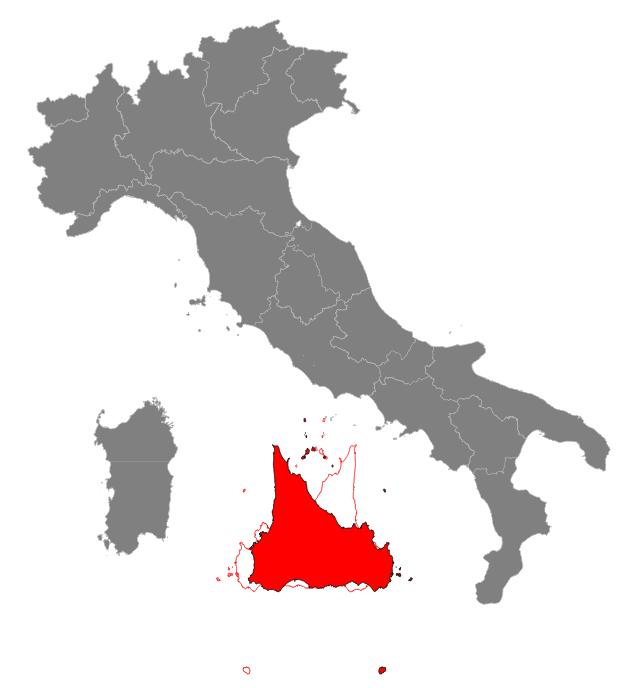### Final considerations and conclusions

1. Agriculture: after applying the suggested relocation Sicily will gain an increased agricultural surface and will enjoy a more favourable climate: still sunny and warm but much less torrid and arid. This will certainly sustain a noticeable development of many economic activities based on agri-food industries.
2. Transportation systems: the suggested new layout for the Lower Tyrrhenian Sea strongly facilitates the development of maritime transports. Sicily could now become the central hub of an efficient network of high-speed and high-frequency ferry connections:
• Palermo will now directly face Naples; Civitavecchia (Rome) seems to be a second obvious terminal for direct connections leading to Central Italy.
• Messina will acquire a decisive strategic role, and will become the terminal for ferry connections leading to Central and Northern Italy: Civitavecchia, Leghorn and Genoa are the obvious destinations.
• A less relevant (but anyway interesting) ferry link will join Trapani and Reggio Calabria.
• Sardinia as well will strongly benefit from the new layout; Cagliari will be directly connected to Syracuse (or may be Augusta), and Olbia to Messina.
This means definitely breaking the secular insulation of Sardinia, that will now start enjoying a stronger and more effective integration with Southern Italy.
• Last but not least: at a more strategic level it's absolutely obvious that now supertankers, big container ships and cruise liners can freely circumnavigate Sicily in any direction under uncompromised safety conditions.
And consequently all Tyrrhenian harbors will now be directly connected both to Eastern and Western Mediterranean: this will surely induce a remarkable growth in the volumes of international traffics they could potentially attract.
3. Heavy industry: the new transportation system strongly centered around maritime communications will surely induce an active rebirth of shipyards, a flourishing traditional excellence of many southern regions in past times but nowadays a sadly declining activity.
4. Tourism: a rearranged Lower Tyrrhenian Sea will certainly become a very attractive destination for international tourists.
It's worth noting that the proposed layout will give birth to a wonderful island group extending between Sicily and southern Lazio - Campania; the Pontine, Aeolian and Gulf of Naples islands will be practically merged into a single archipelago.
Several of these islands actually are active volcanoes, and the new island chain will directly join Mount Etna and Mount Vesuvius.
So this area will represent the most impressive volcanic field of Europe and will certainly become a major tourist attraction thanks to its nicely sunny weather and pleasant climate.
5. Internal commerce: we can easily forecast a strong growth in volume of internal exchanges thanks to the better connectivity based on maritime transports.
Just a single example: Sardinia should now be able to export its finest sheep cheese on Calabrian markets whilst Calabria could freely export its renown red hot chilly peppers to Sardinia; not only both regions will widely benefit from increased exchange volumes, but Sicily as well will take profit from flourishing logistic and by other activities based on commercial intermediation services.
6. Practical realization: the present study clearly demonstrates that there is nothing in Mathematics, Geometry or Geography forbidding the practical realization of the suggested idea.
Unhappily the current state of the art in Geology still poses many puzzling problems not yet fully resolved; anyway we are hopefully expecting that future advancements in Tectonics will possibly allow to overcome any remaining issue.
More specifically a better knowledge of deep interactions between the crust and the upper mantle along the Moho and a more detailed comprehension of the micro-plaques mechanics will certainly help; we are rather confident in future research achievements on these fields.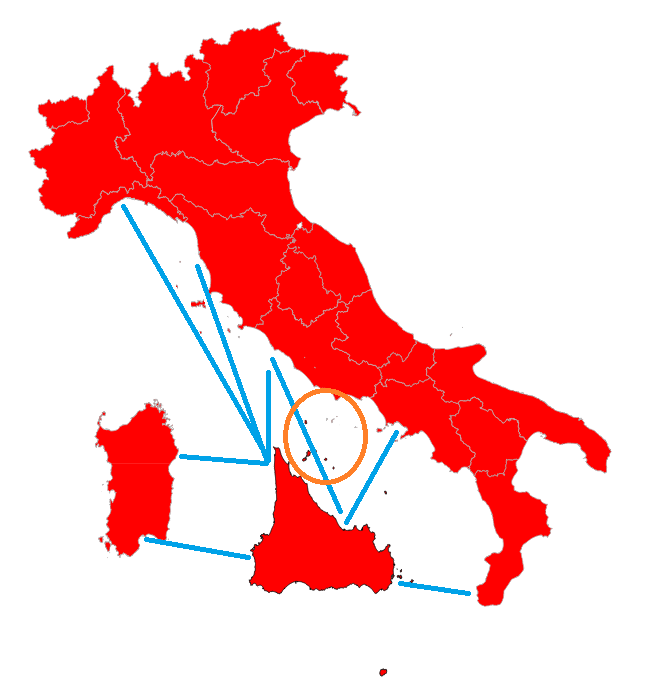## Boring Math: a more formal presentation

Playtime is over: we'll now start a more serious explanation.

An Affine Transformation can be represented in the form of a square matrix; the simpler 2D case requires a 3 x 3 matrix, and the followings are the possible arrangements corresponding to each elementary transformation:

 General layout / a b xoff \ | d e yoff | \ 0 0 1 / Identity / 1 0 0 \ | 0 1 0 | \ 0 0 1 / Translate(tx, ty) / 1 0 tx \ | 0 1 ty | \ 0 0 1 / Scale(sx, sy) / sx 0 0 \ | 0 sy 0 | \ 0 0 1 / Rotate(θ) / cos(θ) -sin(θ) 0 \ | sin(θ) cos(θ) 0 | \ 0 0 1 /

A 3D affine transformation requires a 4 x 4 matrix.
As you can easily notice there is an obvious direct relation between a 3D matrix and a 2D matrix; notice the cells showing a gray background.

 General layout / a b c xoff \ | d e f yoff | | g h i zoff | \ 0 0 0 1 / Identity / 1 0 0 0 \ | 0 1 0 0 | \ 0 0 1 0 / \ 0 0 0 1 / Translate(tx, ty, tz) / 1 0 0 tx \ | 0 1 0 ty | | 0 0 1 tz | \ 0 0 0 1 / Scale(sx, sy, sz) / sx 0 0 0 \ | 0 sy 0 0 | | 0 0 sz 0 | \ 0 0 0 1 / X Roll(θ) / 1 0 0 0 \ | 0 cos(θ) -sin(θ) 0 | | 0 sin(θ) cos(θ) 0 | \ 0 0 0 1 / Y Roll(θ) / cos(θ) 0 sin(θ) 0 \ | 0 1 0 0 | | -sin(θ) 0 cos(θ) 0 | \ 0 0 0 1 / Z Roll(θ) / cos(θ) -sin(θ) 0 0 \ | sin(θ) cos(θ) 0 0 | | 0 0 1 0 | \ 0 0 0 1 /

### applying an Affine Transformation

In order to materialize an affine transformation we simply have to compute (x', y', z') coordinates starting from (x, y, z) for every point or vertex found in the input Geometry accordingly to the following formulae:
• x' = a*x + b*y + c*z + xoff
• y' = d*x + e*y + f*z + yoff
• z' = g*x + h*y + i*z + zoff

in the simpler 2D case this will assume the reduced form:
• x' = a*x + b*y + xoff
• y' = d*x + e*y + yoff

As you can see, applying an Affine Transformation does not requires computing any trigonometric function.
Trigonometric functions are very costly in computational terms, so applying an Affine Transformation is an intrinsically efficient mechanism because it only requires multiplications and additions.

### chaining two (or even more) Affine Transformations in a single operation

Affine transformation matrices have another astonishing property.
We can multiply two different affine transformation matrices thus obtaining a third matrix, and this latest once applied will contain both transformations and in the right sequence.
There is no limit; we can infinitely chain as many transformations as required, we'll simply have to continue multiplying all matrices one after the other carefully respecting the appropriate sequence.
At the end of the process we'll always get just a single affine transformation matrix faithfully representing any individual transformation in the chain.
 The multiplication between two matrices is not a commutative operation: the relative order of operands is absolutely relevant.

### multiplying two matrices

Multiplication is not really a simple operation when matrices are involved and requires a rather complex procedure; the following example shows how to multiply two 4 x 4 matrices.
 / a11 a12 a13 a14 \ | a21 a22 a23 a24 | | a31 a32 a33 a34 | \ a41 a42 a43 a44 /
*
 / b11 b12 b13 b14 \ | b21 b22 b23 b24 | | b31 b32 b33 b34 | \ b41 b42 b43 b44 /
=
 / (a11*b11 + a12*b21 + a13*b31 + a14*b41) (a11*b12 + a12*b22 + a13*b32 + a14*b42) (a11*b13 + a12*b23 + a13*b33 + a14*b43) (a11*b14 + a12*b24 + a13*b34 + a14*b44) \ | (a21*b11 + a22*b21 + a23*b31 + a24*b41) (a21*b12 + a22*b22 + a23*b32 + a24*b42) (a21*b13 + a22*b23 + a23*b33 + a24*b43) (a21*b14 + a22*b24 + a23*b34 + a24*b44) | | (a31*b11 + a32*b21 + a33*b31 + a34*b41) (a31*b12 + a32*b22 + a33*b32 + a34*b42) (a31*b13 + a32*b23 + a33*b33 + a34*b43) (a31*b14 + a32*b24 + a33*b34 + a34*b44) | \ (a41*b11 + a42*b21 + a43*b31 + a44*b41) (a41*b12 + a42*b22 + a43*b32 + a44*b42) (a41*b13 + a42*b23 + a43*b33 + a44*b43) (a41*b14 + a42*b24 + a43*b34 + a44*b44) /

### identity matrix

An identity matrix simply corresponds to an affine transformation lacking any actual effect: at the end of the process the transformed geometry will be exactly the same as before.
Moreover an identity matrix plays a special role in multiplication: the resulting matrix will always be exactly the same of the other matrix.
(it's more or less the equivalent of multiplying e.g. 8*1=8 in an ordinary scalar multiplication).

### inverse matrix

If two (non-identity) matrices are such that their multiplication produces an identity matrix they are said to be one the inverse of the other.
In simpler words both them cause the same identical trasformations but in exactly opposite ways, so that the final combination simply is a no-op.
Please note: not all Affine Trasformation matrices necessarily have a corresponding inverse matrix.

### Boring SQL functions: a formal explanation

SQL FunctionDescription
ATM_Transform ( BLOB Geometry , BLOB AT-matrix ) : BLOB GeometryWill return a new Geometry by applying an Affine Transformation to the input Geometry.
The output Geometry will preserve the original SRID, dimensions and type.
NULL will be returned on invalid arguments.
ATM_Transform ( BLOB Geometry , BLOB AT-matrix , int srid ) : BLOB GeometrySame as above, but the output Geometry will assume the explicitly set SRID.
ATM_IsValid ( BLOB AT-matrix ) : BOOLEANWill check if a BLOB do really correspond to an encoded Affine Transformation Matrix then returning TRUE or FALSE.
-1 will be returned if the argument isn't of the BLOB type.
ATM_AsText ( BLOB AT-matrix ) : TEXTWill return a text serialized representation of the Matrix.
NULL will be returned on invalid arguments.
basic SQL functions on Affine Transformation Matrix
ATM_Multiply ( BLOB AT-matrix-A , BLOB AT-matrix-B ) : BLOB AT-matrixWill multiply Matrix-B by Matrix-A then returning the resulting Matrix.
NULL will be returned on invalid arguments.
ATM_Determinant ( BLOB AT-matrix ) : doubleWill return the determinant of the Matrix.
NULL will be returned on invalid argument.
ATM_IsInvertible ( BLOB AT-matrix ) : booleanWill return 1 TRUE if the Affine Transformation matrix can be inverted, 0 FALSE if not (only matrices presenting a determinant different from zero can be inverted).
NULL will be returned on invalid argument.
ATM_Invert ( BLOB AT-matrix ) : BLOB AT-matrixWill return the inverse matrix.
NULL will be returned on invalid argument.
SQL functions creating and initializing a new Affine Transformation Matrix
ATM_Create ( void ) : BLOB AT-matrixWill return an Identity Affine Transformation Matrix.
NULL if any error occurs.
ATM_Create ( double a , double b , double d , double e , double xoff , double yoff ) : BLOB AT-matrixWill return a 2D Affine Transformation Matrix initialized with explicit values.
NULL if any error occurs or on invalid arguments.
ATM_Create ( double a , double b , double c , double d , double e , double f , double g , double h , double i , double xoff , double yoff , double double zoff ) : BLOB AT-matrixWill return a 3D Affine Transformation Matrix initialized with explicit values.
NULL if any error occurs or on invalid arguments.
ATM_CreateTranslate ( double tx , double ty ) : BLOB AT-matrix
ATM_CreateTranslate ( double tx , double ty , double tz ) : BLOB AT-matrix
Will return an Affine Transformation Matrix initialized respectively as a 2D or 3D Translate.
NULL if any error occurs or on invalid arguments.
ATM_CreateScale ( double sx , double sy ) : BLOB AT-matrix
ATM_CreateScale ( double sx , double sy , double sz ) : BLOB AT-matrix
Will return an Affine Transformation Matrix initialized respectively as a 2D or 3D Scale.
NULL if any error occurs or on invalid arguments..
ATM_CreateRotate ( double angleInDegrees ) : BLOB AT-matrix
ATM_CreateZRoll ( double angleInDegrees ) : BLOB AT-matrix
Will return an Affine Transformation Matrix initialized as Rotation around the Z axis.
NULL if any error occurs or on invalid arguments.
The angle is always expected to be measured in decimal degrees. The direction of rotation is defined such that positive angles rotate in the direction from the positive X axis toward the positive Y axis. With the default axis orientation positive angles rotate in a counterclockwise direction.
Note: this is the unique rotation allowed on a purely 2D plane.
ATM_CreateXRoll ( double angleInDegrees ) : BLOB AT-matrixWill return an Affine Transformation Matrix initialized as Rotation around the X axis.
NULL if any error occurs or on invalid arguments.
ATM_CreateYRoll ( double angleInDegrees ) : BLOB AT-matrixWill return an Affine Transformation Matrix initialized as Rotation around the Y axis.
NULL if any error occurs or on invalid arguments.
SQL functions supporting chaining two Affine Transformation Matrices
ATM_Translate ( BLOB AT-matrix , double tx , double ty ) : BLOB AT-matrix
ATM_Translate ( BLOB AT-matrix , double tx , double ty , double tz ) : BLOB AT-matrix
Will return an Affine Transformation Matrix by chaining respectively a 2D or 3D Translate and another Affine Transformation.
NULL if any error occurs or on invalid arguments.
ATM_Scale ( BLOB AT-matrix , double sx , double sy ) : BLOB AT-matrix
ATM_Scale ( BLOB AT-matrix , double sx , double sy , double sz ) : BLOB AT-matrix
Will return an Affine Transformation Matrix by chaining respectively a 2D or 3D Scale and another Affine Transformation.
NULL if any error occurs or on invalid arguments..
ATM_Rotate ( BLOB AT-matrix , double angleInDegrees ) : BLOB AT-matrix
ATM_ZRoll ( BLOB AT-matrix , double angleInDegrees ) : BLOB AT-matrix
Will return an Affine Transformation Matrix by chaining a Rotation around the Z axis and another Affine Transformation.
NULL if any error occurs or on invalid arguments.
ATM_XRoll ( BLOB AT-matrix , double angleInDegrees ) : BLOB AT-matrixWill return an Affine Transformation Matrix by chaining a Rotation around the X axis and another Affine Transformation.
NULL if any error occurs or on invalid arguments.
ATM_YRoll ( BLOB AT-matrix , double angleInDegrees ) : BLOB AT-matrixWill return an Affine Transformation Matrix by chaining a Rotation around the Y axis and another Affine Transformation.
NULL if any error occurs or on invalid arguments.
Note: all the above functions simply are convenience methods intended to avoid any need to repeatedly call ATM_Multiply().
```SELECT ATM_Multiply(ATM_CreateRotate(15),
ATM_Multiply(ATM_CreateScale(1.1, 1.2, 1.3),
ATM_CreateTranslate(10, 20, 30)));

SELECT ATM_Rotate(
ATM_Scale(
ATM_CreateTranslate(10, 20, 30),
1.1, 1.2, 1.3),
15);

SELECT ATM_Rotate(
ATM_Scale(
ATM_Translate(
ATM_Create(),
10, 20, 30),
1.1, 1.2, 1.3),
15);
```
All three statements will return exactly the same identical Affine Transformation Matrix; anyway the second notation is obviously most concise and more practical than the other two.
Note: in any complex chain of transformations the innermost operation will be applied first, and the outermost operation will be applied last.

Note: the SQL interface supporting Affine Trasformations is designed in such a way that any complexity and difficulty required in order to directly handle matricial operations is completely hidden.
You are simply required to chain several elementary transformations, each one of them basically simple, in the correct sequence and that's absolutely all.
ATM SQL functions will magically and silently keep care of any mathematical complexity.

### Conclusion

• Affine Transformations are a very powerful and very flexible mathematical instrumentation, and their practical utility is noticeably relevant in many applied branches of computing, for example.:
• advanced 2D / 3D graphics
• videogames